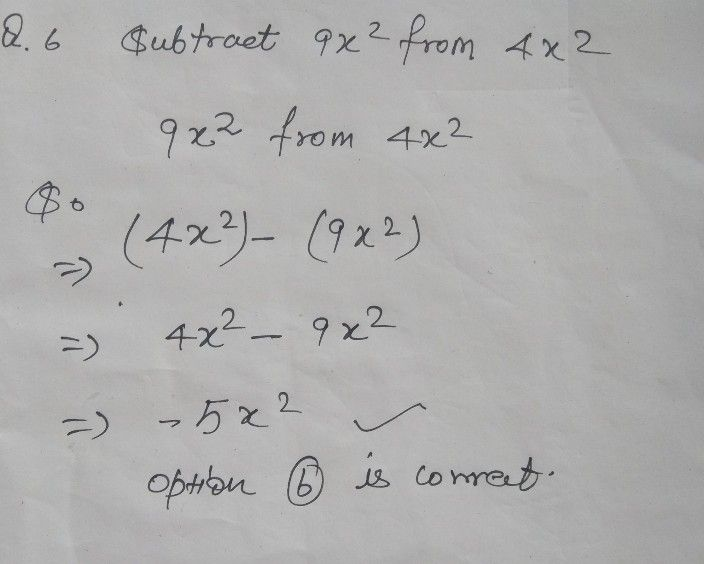Symbol
Problem$Q.6$ Subtract $9\times n_{2}$ from $4\times n_{2}$ $-4xy$ $0$ $0$ $-5\times n2$ $O$ $-6\times n2$ $O$ $5\times n_{2}$